# Mathematics - All About Geometry (Part 6)

in STEMGeekslast year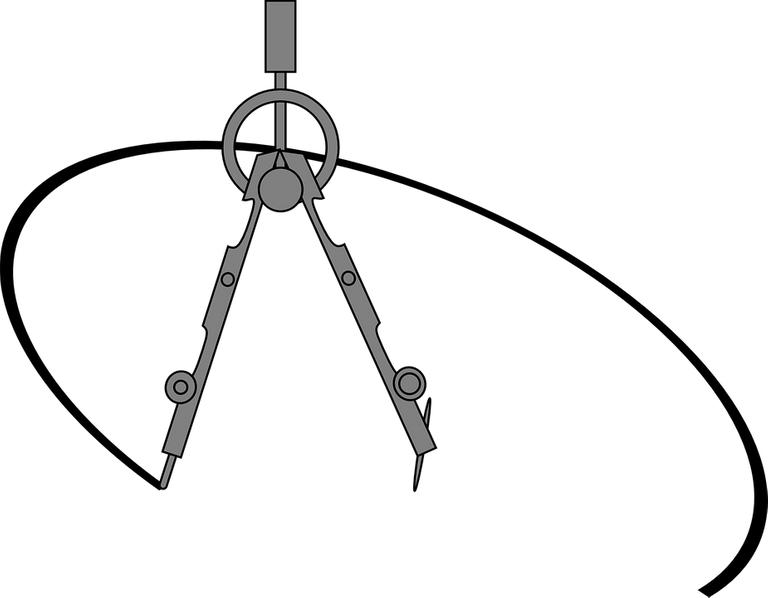[Image1]

## Introduction

Hey it's a me again @drifter1!

This is the sixth and final part of my high-school refresher series on Geometry. I highly suggest checking out part1, part2, part3, part4 and part5 before this part.

So, without further ado, let's get straight into it!

## Analytic Geometry Basics

A point in the Cartesian Coordinate System, the so called x-y plane, is denoted as (x1, y1). This doesn't represent an open interval of numbers of the form (a, b), which is commonly used in mathematics, but a point in this x-y plane. We covered the system more thoroughly in part 3. This is the basis of Analytical Geometry, where mathematical equations are used in order to represent shapes and their relation.

### Delta notion

Let's start simple. Considering two points A and B in this x-y plane, moving from point A to B there will be a change in the x and/or y coordinates. This change is called a delta, Δ (capital Greek alphabet delta). Δx is the change in x, whilst Δy the change in y.

For example, if A = (3, 1) and B = (2, 3) then moving from A to B yields: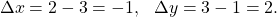The general formulas for points (x1, y1) and (x2, y2) are: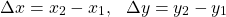### Slope

Having two distinct points A and B, only one straight line can be drawn that passes through both points. This line has a slope equal to the ratio of Δy and Δx or: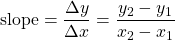For the previous example, we have: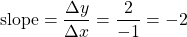### Straight Line

The simplest straight line equation (the slope-intersept equation) is: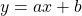where a is the slope.

This equation is basically the result of refactoring the slope equation and entering any point that's on the line, such as: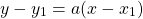This is the so called point-slope equation of a straight line.

For example, in the previous example, entering either of the two points results in the same line, which is: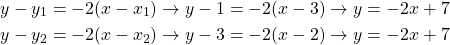The more general formula of a straight line is: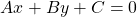where A, B and C are constants, and A, B are not both 0.

Any y = b line is a horizontal line, whilst any x = a line is a vertical line.

### Axes Intersection Points

To find the y value for a specific x value we simply input x into the equation and solve for y (the opposite for finding x). Thus, in order to find where the line intersects the x-axis we set y = 0 and to find where the line intersects the y-axis we set x = 0. Simple stuff.

For example, for the previous line x = 0 and y = 0 yield the following intersection points: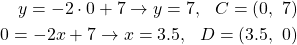### Parallel Lines

Two lines are parallel to each other if the slopes are equal.

For example: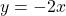is a line parallel to the previous example's line.

### Perpendicular Lines

Two lines are parallel if their slopes are negative reciprocales: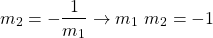For example: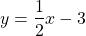is a line perpendicular to the previous example's line as: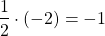### Line Intersection Point

In order to find where two or more lines meet (if they meet) their linear system needs to be solved which is a topic covered by Linear Algebra.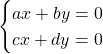For example: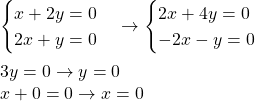### Distance Between 2 Points

The distance between two points (x1, y1) and (x2, y2) is a direct consequence of Pythagoras's Theorem and equal to: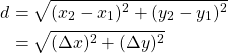For example, the distance between the points A and B from before is: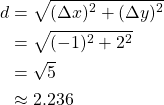### Midpoints

Considering the line segment which joins two points (x1, y1) and (x2, y2), the midpoint of this segment is at: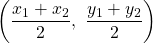### Conics

The simplest non-straight lines are the conics covered by the end of part 4.

Their general equation is of the form: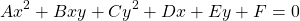The Bxy term is about conic rotation. The Dx, Ey and F terms affect the vertex and center points. And lastly, for A, C we have the following shapes:

• A = C : Circle
• AC > 0 (A ≠ C) : Ellipse
• AC = 0 : Parabola
• AC < 0 : Hyperbola

For example, a circle with center at (2, -2) and radius of 2, has the following equation: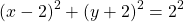which can also be expanded into the general equation format: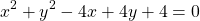These are all topics of the branch of mathematics known as Mathematical Analysis, which also deals with topics such as limits, differentiation, integration, sequences, series etc.

## RESOURCES:

### Images

Block diagrams and other visualizations were made using draw.io.

## Final words | Next up

And this is actually it for today's post and this small refresher series!